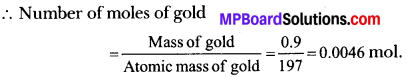## MP Board Class 9th Science Solutions Chapter 3 Atoms and Molecules

### Atoms and Molecules Intext Questions

Atoms and Molecules Intext Questions Page No. 32 – 33

Question 1.
In a reaction 5.3g of sodium carbonate reacted with 6g of ethanoic acid. The products were 2.2g of carbon dioxide. 0. 9g water and 8.2g of sodium ethanoate. Show that these observations are in agreement with the law of co serration of mass.
Sodium carbonate + ethanoic acid → sodium ethanoate + carbon dioxide + water
The reaction is,
Sodium carbonate + ethanoic acid → sodium ethanoate + carbon dioxide + water
5.3g + 6g → 8.2g + 2.2g + 0.9g
Now,
Total mass of reactants = (5.3 + 6)g = 11.3g
And, total mass of products = (8.2 + 2.2 + 0.9)g = 11.39g
So, Mass of reactants = Mass of product
It shows the law of conservation of mass.Question 2.
Hydrogen and oxygen combine in the ratio of 1 : 8 by mass to form water. What mass of oxygen gas would be required to react completely with 3g of hydrogen gas?
Ratio of hydrogen and oxygen in water = 1 : 8
So, oxygen is 8 times that of hydrogen by mass.
Let, xgrams of oxygen will react with 3g of hydrogen.
Then,
1 : 8 = 3 : x
x = 8 × 3
x = 24g
∴ 24g of oxygen gas required.

Question 3.
Which postulate of Dalton’s atomic theory is the result of the law of conservation of mass?
Postulate of Dalton’s theory based on law of conservation of mass is “Atoms are indivisible particles which can neither be created nor be destroyed in a chemical reaction.”

Question 4.
Which postulate of Dalton’s atomic theory can explain the law of definite proportions?
Postulate is the relative number and kinds of atoms remain constant in a given compound.

Atoms and Molecules Intext Questions Page No. 35

Question 1.
Define the atomic mass unit.
Atomic mass unit is the mass unit equal to $$\frac { 1 }{ 12 }$$th mass of one carbon-12 atom.

Question 2.
Why is it not possible to see an atom with naked eyes?
The size of an atom is very very small that we can see with naked eyes. The size of an atoms lies in nano meters (nm).

Atoms and Molecules Intext Questions Page No. 39

Question 1.
Write down the formulae of:
(i) Sodium oxide
(ii) Aluminium chloride
(iii) Sodium sulphide
(iv) Magnesium hydroxide.
(i) Sodium Oxide

• Symbol → NaO
• Charge → +1-2
• Formula → Na2O

(ii) Aluminium Chloride

• Symbol → AlCl
• Charge → +3-1
• Formula → AlCl3

(iii) Sodium Sulphate

• Symbol → NaS
• Charge → +1-2
• Formula → Na2S

(iv) Magnesium Hydroxide

• Symbol → MgOH
• Charge → +2-1
• Formula → Mg(OH)2Question 2.
Write down the names of compounds represented by the following formulae:

1. Al2(SO4)3
2. CaCl2
3. K2SO4
4. KNO3
5. CaCO3

1. Al2(SO4)3 → Aluminium sulphate
2. CaCl2 → Calcium chloride
3. K2SO4 → Potassium sulphate
4. KNO3 → Potassium nitrate
5. CaCO3 → Calcium carbonate

Question 3.
What is meant by the term chemical formula?
It is the representation of composition of a compounds in the form of symbols of elements present in it.

Question 4.
How many atoms are present in a:

1. H2S molecule and
2. PO43- ion?

1. H2S Molecule -2 atoms of H + 1 atom of S = Total 3 atoms.
2. PO43- 1 atom of phosphorus + 4 atoms of oxygen total 5 atoms.

Atoms and Molecules Intext Questions Page No. 40

Question 1.
Calculate the molecular masses of:

1. H2
2. O2
3. Cl2
4. CO2
5. CH4
6. C2H6
7. C2H4
8. NH3
9. CH3OH.

1. H2 = (2 × 1)u = 2u
2. O2 = (2 × 16)u = 32u
3. Cl2 = (2 × 35.5)u = 71u
4. CO2 = (1 × 12 + 2 × 16)u = (12 + 32)u = 44u
5. CH4 = (1 × 12 + 4 × 1)u = (12 + 4)u = 16u
6. C2H6 = (2 × 12 + 6 × 1)u = (24 + 6)u = 30u
7. C2H4= (2 × 12 + 4 × 1)u = (24 + 4)u = 28u
8. NH3 = (1 × 14 + 3 × 1)u = (14 + 3)u = 17u
9. CH3OH = (1 × 12 + 3 × 1 + 1 × 16 + 1 × 1)u = (12 + 3 + 16 + 1)u = 32u.

Question 2.
Calculate the formula unit masses of ZnO, Na2O, K2CO3, given atomic masses of Zn = 65u, Na = 23u, K = 39u, C = 12u and O = 16u.
Formula unit mass of

1. ZnO = (1 × 65 + 1 × 16)u = (65 + 16)u = 81u
2. Na2O = (2 × 23 + 1 × 16)u = (46 + 16)u = 62u
3. K2CO3 = (2× 39 + 1 × 12 + 3 × 6)u = (78 + 12 + 48)u = 138u

Atoms and Molecules Intext Questions Page No. 42

Question 1.
If one mole of carbon atoms weighs 12 gram, what is the mass (in gram) of 1 atom of carbon?
1 mole of carbon atoms = 6.022 × 1023 atoms
Also, 1 mole of carbon atoms = 12g
6.022 × 1023 atoms of carbon weigh = 12g
1 atom of carbon weigh = 1.99 × 1023g.

Question 2.
Which has more number of atoms, 100 grams of sodium or 100 grams of ion (given atomic mass of Na = 23u, Fe = 56u)?
1 mole of sodium = 23g of Na
Atoms = 6.022 × 1023 atoms
23g of Na = 6.022 × 1023 atoms
100g of Na = $$\frac { 100 }{ 23 }$$ × 6.022 × 1023 = 2.617 × 1024 atoms 8 23
Now, 1 mole of iron atoms = 56g of Fe = 6.022 × 1023 atoms
56g of Fe = 6.022 × 1023 atoms
100g of Fe = $$\frac { 100 }{ 56 }$$ × 6.022 × 1023 = 1.075 × 1024 atoms
So, 100 g of Na contains more atoms.

### Atoms and Molecules NCERT Textbook Exercises

Question 1.
A 0.24 g sample of compound of oxygen and boron was found by analysis to contain 0.096g of boron and 0.144g of oxygen. Calculate the percentage composition of the compound by weight.
Percentage composition of boron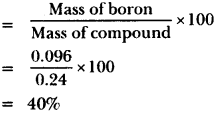Percentage composition of oxygen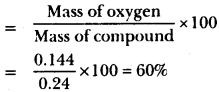Question 2.
When 3.0g of carbon is burnt in 8.00g oxygen, 11.00g of carbon dioxide is produced. What mass of carbon dioxide will be formed when 3.00g of carbon is burnt in 50.00g of oxygen? Which law of chemical combination will govern your answer?
We know that 3g of carbon is burnt in 8g of oxygen to form 11g of carbon dioxide.
When 3g of carbon is burnt in 50g of oxygen, then 11g of carbon dioxide will be formed.
And oxygen remain unreacted, (50 – 8)g = 42g.
Law of constant proportion governs here.

Question 3.
What are polyatomic ions? Give examples.
The ions which contain more than one type of atoms (same kind or different kinds) as a single unit are called polyatomic ions.
Examples:

• Sulphate ion (SO4-2)
• Nitrate ion (NO3-2)
• Carbonate ion (CO3-2).

Question 4.
Write the chemical formulae for the following:
(a) Magnesium chloride
(b) Calcium oxide
(c) Copper nitrate
(d) Aluminium chloride
(e) Calcium carbonate.
(a) Magnesium chloride – MgCl2
(b) Calcium oxide – CaO
(c) Copper nitrate – Cu(NO3)2
(d) Aluminium chloride – AlCl3
(e) Calcium carbonate – CaCO3Question 5.
Give the names of the elements present in the following compounds:
(a) Quick lime
(b) Hydrogen bromide
(c) Baking powder
(d) Potassium sulphate
(a) Quick lime (calcium oxide): Elements: Calcium and oxygen.
(b) Hydrogen bromide: Elements: Hydrogen and bromide.
(c) Baking powder (sodium hydrogen carbonate) Elements: Sodium, hydrogen, carbon, oxygen.
(d) Potassium sulphate: Elements: Potassium, sulphur, oxygen.

Question 6.
Calculate the molar mass of the following substances:
(a) Ethyne, C2H2
(b) Sulphur molecule, S8
(c) Phosphorus molecule, P4 (Atomic mass of phosphorus = 31)
(d) Hydrochloric acid, HCl
(e) Nitric acid, HNO3.
(a) Ethyne, C2H2 = (2 × 12 + 2 × 1)g = (24 + 2)g = 26g
(b) Sulphur molecules, S8 = (8 × 32)g = 256g
(c) Phosphorus molecule, P4 = (4 × 31)g = 124g
(d) Hydrochloric acid, HCl = (1 × 1 + 1 × 35.5)g = (1 + 35.5)g = 36.5g
(e) Nitric acid, HNO3 = (1 × 1 + 1 × 14 + 3 × 16)g = (1 + 14 + 48)g = 63g

Question 7.
What is the mass of:
(a) 1 mole of nitrogen atoms?
(b) 4 moles of aluminium atoms (Atomic mass of aluminium = 27)?
(c) 10 moles of sodium sulphite (Na2SO3)?
(a) 1 Mole of nitrogen atoms = 14g.

(b) Mass of 1 mole of aluminium atoms = 27g.
So, mass of 4 moles of aluminium atoms = (4 × 24)g = 108g.

(c) Mass of 1 mole of sodium sulphide
= (2 × 23 + 32 × 1 + 3 × 16)g = (46 + 32 + 48)g = 126g
∴ Mass of 10 moles of Na2SO3 = (126 × 10)g = 1260g.

Question 8.
Convert into mole:
(a) 12g of oxygen gas
(b) 20g of water
(c) 22g of carbon dioxide.
(a) Mass of oxygen gas = 12g
Now, 1 mole of oxygen gas = 32g moles mass
So, 32g molar mass of oxygen = 1 moles
∴ 1g of oxygen gas = $$\frac { 1 }{ 32 }$$ moles
and 12 g of oxygen gas = $$\frac { 1 }{ 32 }$$ moles × 12 moles = 0.375 moles.

(b) 1 Mole of water = 18g molar mass
So, 18g moles mass of water = 1 mole
∴ 1g of water = $$\frac { 1 }{ 18 }$$ moles
and 20 g of water = $$\frac { 1 }{ 18 }$$ moles × 20 moles = 1.11 moles.

(c) 1 Mole of carbon dioxide = 44g molar mass
So, 44g molar mass of = 1 mole carbon dioxide
∴ 22g of carbon dioxide = $$\frac { 22 }{ 44 }$$ moles = 0.5 mole.Question 9.
What is the mass of
(a) 0.2 mole of oxygen atoms?
(b) 0.5 mole of water molecules?
(a) 1 mole of oxygen atoms = 16g
∴ 0.2 moles of oxygen atoms = (16 × 0.2)g = 3.2g

(b) 1 mole of water molecules = 18g
∴ 0.5 mole of water molecule = (18 × 0.5)g = 9g.

Question 10.
Calculate the number of molecules of sulphur (S8) present in 16g of solid sulphur.
256g of sulphur = 1 mole of sulphur molecules
So, 16g of sulphur = $$\frac { 1 }{ 16 }$$ × 16 moles of sulphur molecules
= 0.0625 mole of sulphur molecule
Also, 1 mole of sulphur molecules = 6.023 × 1023 molecules
So, 0.0625 moles of sulphur molecules = 6.025 × 1023 × 0.0625 molecules
= 3.76 × 1022 molecules.

Question 11.
Calculate the number of aluminium ions present in 0.051g of aluminium oxide.
(Hint: The mass of an ion is the same as that of an atom of the same element. Atomic mass of Al = 27u).
Molar mass of aluminium oxide (Al2O3) = (2 × 27)g + (3 × 16)g
= (54 + 48)g = 102g
∴ 102g of aluminium = 6.022 × 1023 oxide contains aluminium ions
So, 0.051g Al2O3 contains aluminium ions = 6.022 × 1020 aluminium ions.

### Atoms and Molecules Additional Questions

Atoms and Molecules Multiple Choice Questions

Question 1.
Atomic theory of matter was proposed by.
(a) Newton
(b) John Dalton
(c) Rutherford
(d) Lavoisier.
(b) John Dalton

Question 2.
Law of Conservation of mass was given by.
(a) Lavoisier
(b) Dalton
(c) Kennedy
(a) Lavoisier

Question 3.
The term mole was first introduced by.
(a) Grahm
(b) Dalton
(c) Ostwald
(d) Boyle.
(c) Ostwald

Question 4.
Atomic radius of an atom is measured in.
(a) Micrometre
(b) Millimetre
(c) Nanometre
(d) Centimetre.
(c) Nanometre

Question 5.
Law of constant proportions was proposed by.
(a) Dalton
(b) Bezelius
(c) Proust
(d) Lavoisier.
(c) Proust

Question 6.
Latin name of an atom is argentum. The English name of this element is.
(a) Argon
(b) Gold
(c) Silver
(d) Mercury.
(c) Silver

Question 7.
Phosphorus molecule is.
(a) Diatomic
(b) Triatomic
(c) Tetra – atomic
(d) Mono – atomic.
(c) Tetra – atomicQuestion 8.
The atom chosen for reference for measuring atomic masses is.
(a) C-14
(b) C-12
(c) H-2
(d) O-12.
(b) C-12

Question 9.
IUPAC is.
(a) Indian Union of Pacific and Applied Chemistry.
(b) International Union of Permanent and Applied Chemicals.
(c) International Union of Pure and Applied Chemistry.
(d) Indian Union of Pure and Applied Chemistry.
(c) International Union of Pure and Applied Chemistry.

Question 10.
Atomic mass is measured in.
(a) Grams
(c) Centigrams
(d) Atomic mass unit.
(d) Atomic mass unit.

Question 11.
1 A.M.U. is equal to.
(a) 1.65 × 10-23g
(b) 1.63 × 10-25g
(c) 1.66 × 10-24g
(d) 1.66 × 10-22g.
(c) 1.66 × 10-24g

Question 12.
The naming of elements from first or first and second letter was introduced by.
(a) Berzellius
(b) Dalton
(c) Proust
(d) Lavoisier.
(a) Berzellius

Question 13.
The combining capacity of an element is called.
(a) Atomicity
(b) Valency
(c) Reactivity
(d) None of these.
(b) Valency

Question 14.
The cation of element has.
(a) More electrons than normal atom
(b) Equal electrons than normal atom
(c) Less electrons than normal atom
(d) Equal proton than normal atom.
(c) Less electrons than normal atom

Question 15.
The formula of a compound is A5B4. Then valency of A and B will be.
(a) 5 and 4
(b) 5 and 9
(c) 4 and 9
(d) 4 and 5.
(d) 4 and 5.

Question 16.
1 Mole has.
(a) 6.012 × 1023 particles
(b) 6.022 × 1023 particles
(c) 6.022 × 1022 particles
(d) 6.022 × 1025 particles
(b) 6.022 × 1023 particles

Question 17.
Which of the following has the maximum number of atoms?
(a) 18g of CH4
(b) 18g of H4O
(c) 18g of CO2
(d) 18g of O2.
(a) 18g of CH4

Question 18.
Atomic mass of C6H12O6 is.
(a) 24
(b) 80
(c) 100
(d) 12
(a) 24

Question 19.
1 atomic mass unit is equal to.
a) $$\frac { 1 }{ 14 }$$ mass of a C – 12 atom
(b) $$\frac { 1 }{ 16 }$$mass of a C – 12 atom
(c) $$\frac { 1 }{ 18 }$$ mass of a C – 12 atom
(d) $$\frac { 1 }{ 12 }$$mass of a C – 12 atom.
(d) $$\frac { 1 }{ 22 }$$mass of a C – 12 atom.

Question 20.
Atomicity of Sulphate (SO42-) ion.
(a) Mono – atomic
(b) Tri – atomic
(c) Poly – atomic
(d) Tetra – atomic
(c) Poly – atomic

Question 21.
Formula of number of moles of a substance is.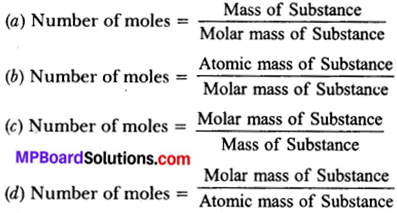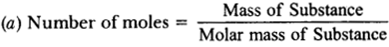Atoms and Molecules Very Short Answer Type Questions

Question 1.
Name the scientist who gave atomic theory of matter.
John Dalton.

Question 2.
Name the scientist who gave the law of conservation of mass.
Antoine LavoisierQuestion 3.
Name the scientist who gave the law of constant proportions.
Joseph Proust.

Question 4.
How many metres are in 1nm?
1nm = 10-9m.

Question 5.
Give two examples of polyatomic molecules of elements.
P4 (Phosphorus) and S8 (Sulphur).

Question 6.
Write full form of IUPAC.
International Union of Pure and Applied Chemistry.

Question 7.
An element A and B has valency of 3 and 4. Write its chemical formula.
A4B3.

Question 8.
Name the scientist who introduced the term ‘mole’.
Wilhem Ostwald.

Question 9.
How many particles exist in 1 mole of atom?
1 mole = 6.022 × 1023 particles.

Question 10.
Write the formula of aluminium sulphate.
Al2(SO4)3.

Question 11.
Name the charged particles formed by gaining of electrons.
Anion.

Question 12.
Name the charged particles formed by loosening of electrons.
Cation.

Question 13.
6.022 × 1023.Question 14.
Write the Latin names of iron, gold and copper.

1. Iron – Ferrum
2. Gold – Aurum
3. Copper – Cuprum.

Question 15.
What is 1 amu?
1 amu = $$\frac { 1 }{ 2 }$$th mass of a carbon-12 atom.

Question 16.
Calculate the number of atoms of oxygen present in its 3.5 moles.
1 mole of oxygen atoms = 6.022 × 1023
∴ 3.5 moles of oxygen atoms = 3.5 × 6.022 × 1023 atoms
= 2.10 × 1024 atoms.

Atoms and Molecules Short Answer Type Questions

Question 1.
Define:
(a) Law of conservation of mass.
(b) Law of constant proportions.
(c) Atomic mass.
(d) Molecules of element.
(e) Molecules of compound.
(f) Atomicity.
(g) Ion.
(h) Chemical formula.
(i) Valency.
(j) Molecular mass.
(k) Formula unit mass.
(l) Mole.
(a) Law of conservation of mass: Matter is neither created nor destroyed during a chemical reaction i.e., total mass of reactants is equal to the total mass of products in a chemical reaction.

(b) Law of constant proportions: In a chemical compound, the elements are always present in a definite proportion by mass.
e.g.:

• In CO2 the ratio of mass of carbon to the mass of oxygen is always 7 : 16.

(c) Atomic mass: The Atomic mass of an element is defined as the relative mass of its atom as compared with the mass of a carbon 12 atom taken as 12 units.

(d) Molecules of element: It contain two or more similar kinds of atoms chemically combined together.
e.g.:

• O2, N2, P4.

(e) Molecules of compound: It contains two or more different kinds of atoms chemically combined together.
e.g.:

• SO4, CO2, H2O.

(f) Atomicity: It is the total number of atoms present in a molecule.
e.g.:

• Atomicity of H2 is 2
• Atomicity of P4 is 4 and
• Atomicity of CO2 is 3.

(g) Ion: It is the positively or negatively charged atom or group of atoms. It is formed by either loosening or gaining of electrons. Positively charged ion is called cation and negatively charged ion is called anion.
e.g.:

• Cation – Na+, Mg2+, NH4+
• Anion – Cl, Br,OH, SO42-

(h) Chemical formula: Chemical formula of a compound is representation in the form of symbols of elements present in it.
e.g.:

• Sodium chloride (NaCl)
• Calcium oxide (CaO).

(i) Valency: It is the combining power (or capacity) atom or group of atoms. It tells number of electrons lost or gained by the atom during the Chemical reaction.
e.g.: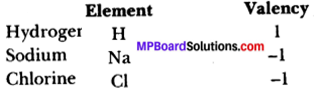(j) Molecular mass: It is the sum of atomic masses of all the atoms in a molecule of the substance.
e.g.:
Molecular mass of CO2 is = Atomic mass of C + 2 × atomic mass of O
= 12 + 2 × 16
= 12 + 32 = 44u
It is expressed in atomic mass unit (u).

(k) Formula unit mass: It is the sum of atomic masses of all atoms in a formula unit of the compound containing constituents as ions. It is also expressed in atomic mass unit (u).
e.g.:
Formula unit mass of NaCl = Atomic mass of Na + atomic mass of Cl.
= (1 × 23 + 1 × 35.5)u
= (23 + 35.5)u = 58.5u.

(l) Mole: One mole of any substance like atoms or molecules or ions is that quantity which has mass equal to its atomic or molecular mass in grams and also contains 6.022 x 1023 particles of that substance.
e.g.:
1 mole of H2 molecule = (1 × 2)g = 2g and also,
1 Mole of H2 molecule = 6.022 × 1023 molecules of hydrogen.Question 2.
Write the postulates of Dalton’s atomic theory.
Postulates:

1. All matter is made up of very tiny particles called atoms.
2. Atoms are indivisible.
3. Atoms can neither be created nor be destroyed in a chemical reaction.
4. Atoms of a given element are identical in mass and chemical properties.
5. Atoms combine in the ratio of small whole numbers to form compounds.
6. The number of atoms and kind of atoms is fixed in a given compound.

Question 3.
What were the drawbacks of Dalton’s atomic theory?
Drawbacks:

1. According to theory, atoms were indivisible but they can be divided in ions in electrons, protons and neutrons under special conditions.
2. According to theory, atoms of an element have masses but it is found that atoms of some elements can have some different masses.
3. According to theory, atoms of different elements have different masses but it is found that atoms of different elements can have same masses.

Question 4.
Calcium carbonate decomposes on heating to form calcium oxide and carbon dioxide. When 20g calcium carbonate is decomposed completely then 11.2g of calcium oxide is formed. Calculate the mass of carbon dioxide formed. Which law of chemical combination will be applied there?
Law of conservation of mass is applied here.
Now,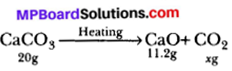Let, x grams of CO2 is formed
Now, according to law of conservation of mass.
Total mass of reactants = Total mass of product
⇒ 20 = 11.2 + x
⇒ x = (20 – 11.2) = 8.89
So, Mass of CO2 formed is 8.8g.

Question 5.
In an experiment 9.8g of copper oxide was obtained from 7.84g of copper. In another experiment 9.1g of copper oxide was obtained on reduction 7.28g of copper. Show with the help of calculations that these figures verify the law of constant proportions.
Case I:
Mass of copper oxide = 9.8g
Mass of copper = 7.84g
Mass = (9.8 – 7.84)g = 1.96g
So, Ratio of copper to oxygen = $$\frac { 7.84 }{ 1.96 }$$ = $$\frac { 4 }{ 1 }$$ = 4 : 1

Case II:
Mass of copper oxide = 9.1g
Mass of copper = 7.28g
∴ Mass of oxygen = (9.1 – 7.28)g = 1.82g
So, Ratio of copper to oxygen = $$\frac { 7.28 }{ 1.82 }$$ = $$\frac { 4 }{ 1 }$$ = 4 : 1
Since, ratio of copper and oxygen in the two samples is same.
The law of constant proportions is verified.

Question 6.
Write the atomicity of following compounds.
(a) H2O
(b) CaCO3
(c) H2SO4
(d) C6H12O6
(e) S8.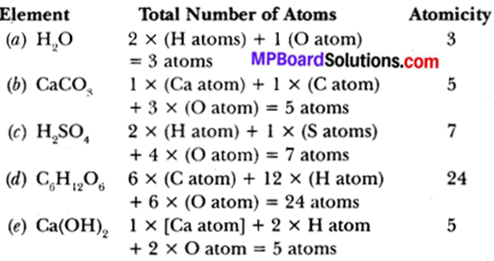Question 7.
Write the chemical formula of:
(a) Hydrogen oxide
(b) Calcium carbonate
(c) Magnesium chloride
(d) Aluminium sulphate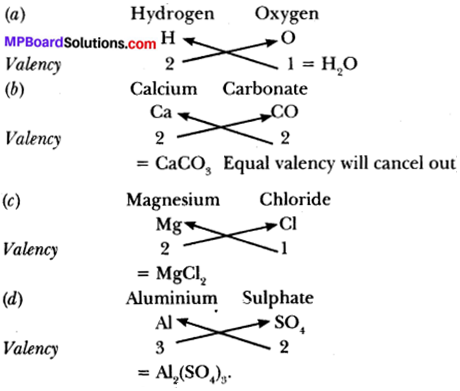Question 8.
Calculate the molecular ma
(a) CH3OH
(b) C6H12O6
(c) NH3
(d) H2SO4
Atomic masses:
C = 12u
H = 1u
O = 16u
N = 14u
S = 32u
(a) Molecular mass of
CH3OH = (1 × 12 + 3 × 1 + 1 × 16 + 1 × 1)u
= (12 + 3 + 16 + 1 )u = 32u.

(b) Molecular mass of
C6H12O6 = (6 × 12 + 12 × 1 + 6 × 16)u
= (72 + 12 + 96)u = 180u.

(c) Molecular mass of
NH3 = (1 × 14 + 3 × 1)u
= (14 + 3)u = 17u.

(d) Molecular mass of
H2SO4 = (1 × 2 + 1 × 32 + 4 × 16)u
= (2 + 32 + 64)u
= 98u.

Question 9.
Calculate the number of molecules of:
(a) SO2 in its 3 moles.
(b) O2 in its 2.5 moles.
(a) 1 mole of SO2 molecule
= 6.022 × 1023 molecules of SO4
= 3 moles of SO4 molecule
= 3 × 6.022 × 1023 molecules
= 18.066 × 1023 molecules.

(b) 1 mole of O2 molecule
= 6.022 × 1023 molecules of O2
= 2.5 moles of O2 molecule
= 2.5 × 6.022 × 1023 molecules of O2
= 15.055 × 1023 molecules
= 1.5055 × 1024 molecules.Question 10.
Calculate the number of moles in:
(a) 12.044 × 1023 molecules of CO2.
(b) 24.012 × 1024 molecules of H2
(a) 1 mole of CO2 = 6.022 × 1023 particles of CO2.
⇒ 6.022 × 1023 particles = 1 mole of CO2.
So, 1 particle of CO2 molecule
= 1 / 6.022 × 1023 mole and,
12.044 × 1023 particles of CO2 molecules
= 1 / 6.022 × 1023 × 12.044 × 1023
= 12.044 × 1023 × 1 / 6.022 × 1023 moles = 2 moles.

(b) 1 mole of H2 contains = 6.022 × 1023 particles of H2 molecule
or 6.022 × 1023 particles of H2 = 1 mole of H2
1 particle of H2 molecule = 1 / 6.022 × 1023 mole of H2.
∴ 24.012 × 1023 particles of H2 = 1 / 6.022 × 1023 × 24.012 × 1023 moles of H2
= 3.98 moles of H2.

Atoms and Molecules Long Answer Type Questions

Question 1.
Calculate the ratio of following elements by mass in the given compound:
(a) Hydrogen and oxygen in water (H2O).
(b) Carbon and oxygen in carbon dioxide (CO2).
(c) Carbon and hydrogen in ethene (C2H4).
(a) In H2O molecule,
Mass of H = 2 × 1g = 2g
Mass of O = 1 × 16g = 16g
So, Ratio of hydrogen and oxygen by mass = $$\frac { 2 }{ 16 }$$ =$$\frac { 1 }{ 8 }$$ = 3 : 8

(b) In CO2 molecule,
Mass of C= 1 × 12g = 12g
Mass of O = 2 × 16g = 32g
.;. Ratio of carbon and oxygen by mass = $$\frac { 12 }{ 32 }$$ = 3 : 8

(c) In ethene (C2H4) molecule,
Mass of C = 2 × 12g = 24g
Mass of H = (4 × 1)g = 4g
Ratio of carbon and hydrogen by mass = $$\frac { 24 }{ 4 }$$ = 6 : 1

Question 2.
Calculate the formula unit mass of the following Ionic Compounds:
(a) Sodium Chloride (NaCl)
(b) Calcium Oxide (CaO)
(c) Copper Sulphate (CuSO4)
(d) Calcium Nitrate [Ca(NO3)2]
[Atomic masses: Na = 23u, Cl = 35.5u, Ca = 40u, O = 16u, Cu = 63.5u, S = 32u, N = 14u]
(a) Formula unit mass of NaCl Molecule = (1 × 23 + 1 × 35.5)u
= (23 + 35.5)u = 58.5u.

(b) Formula unit mass of CaO molecule = (1 × 40 + 1 × 16)u
= (40 + 16)u = 56u.

(c) Formula unit mass of CuSO4 = (1 × 63.5 + 1 × 32 + 4 × 16)u
= (63.5 + 32 + 64)u = 159.5u.

(d) Formula unit mass of [Ca(NO3)2]
= 1 × 40 + 2 [1 × 14 4 + 3 × 16]
= 40 + 2 [14 4 + 48]u
= (40 + 124)u
= 164u.
MOLE CONCEPT (Graus to uoles):

Question 3.
Find the number of moles in:
(a) 20g of H2O
(b) 140g of CO2
(c) 200g of CaCO3.
(a) 1 mole of H2O = Molar mass of H2O
= (2 × 1 + 16)g
So, 1 Mole = 18g of H2O
or 18g of H2O = 1 Mole
1g of H2O = $$\frac { 1 }{ 18 }$$ Mole
and, 20g of H2O = $$\frac { 1 }{ 18 }$$ × 20 = 1.11 Mole

(b) 1 mole of CO2 = Molar mass of CO2 = (1 × 12 + 2 × 16)g
= (12 + 32)g = 44g
So, 1 mole = 44g of CO2
or 44g of CO2 = 1 mole
1 g of CO2 = $$\frac { 1 }{ 44 }$$ mole
and, 140 g of CO2 = $$\frac { 1 }{ 44 }$$ × 140 moles
= 3.18 moles.

(c) 1 mole of CaCO3 = Molar mass of CaCO3
= (1 × 40 + 1 × 12 + 3 × 16)g
= (40 + 12 + 48)g = 100g
So, 1 mole = 100g of CaCO3
or 100g of CaCO3 = 1 mole
1g of CaCO3 = $$\frac { 1 }{ 100 }$$ mole
and, 200 g of CaCO3 = $$\frac { 1 }{ 100 }$$ × 200 moles = 2 moles.
Moles to Grams

Question 4.
Calculate the mass in grams of:
(а) 3 moles of H2O
(b) 2 moles of H2SO4
(c) 1.5 moles of carbon atoms (C atom).

(a) 1 mole of H2O = Molar mass of H2O
= (2 × 1 + 16)g of H2O
= 18g of H2O
∴ 3 mole of H2O = (3 × 18)g of H2O
H2O = 54g of H2O

(b)1 mole of H2SO4 = Molar mass of H2SO4
= (2 × 1 + 1 × 32 + 4 × 16)g = 98g
2 mole of H2SO4 = (2 × 98)g = 196g

(c) 1 mole of C atom = Molar mass of C atom = 12g
∴ 1.5 moles of C -atom = (1.5 × 12)g = 18g.
Moles to Number of Particles.

Question 5.
Calculate the number of particles in:
(a) 115g of H2O.
(b) 60g of CO2.
(a) We know,
1 mole of H2O = molar mass of H2O
= (2 × 1 + 16)g = 18g
It means, 18g of H2O = 1 mole of CO2
1g of H2O = $$\frac { 1 }{ 18 }$$ mole = 6.38 moles
Now, 1 mole of H2O = 6.022 × 1023 particles
6.38 moles of H2O = 6.38 x 6.022 × 1023 particles
= 3.84 × 1024 particles.

(b) We know,
1 mole of CO2 = Molar mass of CO2
= (12 + 2 × 16)g = (12 + 32)g = 44g
It means, 44g of CO2 = 1 mole of CO2
1g of CO2= $$\frac { 1 }{ 44 }$$ mole of CO2
and, 60g of CO2 = $$\frac { 1 }{ 44 }$$ × 60 moles of CO2
= 1.3636 moles
Number of Particles to Mass.

Question 6.
Calculate the mass of:
(a) O2 in its 36.48 × 1025 particles.
(b) NH3 in its 4.012 × 1024 particles.
(a) We know,
1 mole of O2 = 6.023 × 1023 particles
or 6.023 × 1023 particles = 1 mole of O2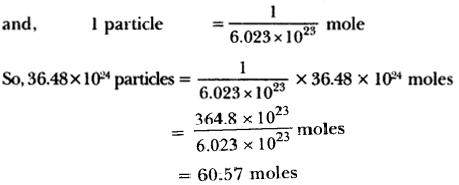Now, We also know,
1 mole of O2 contains = molar mass of O2
So, 60.57 moles of O2 contains = 60.57 × 32g = 1938.49g.

(b) We know,
1 mole of ammonia (NH3) = 6.022 X 1023 particles
or 6.022 × 1023 particles of ammonia (NH3) = 1 mole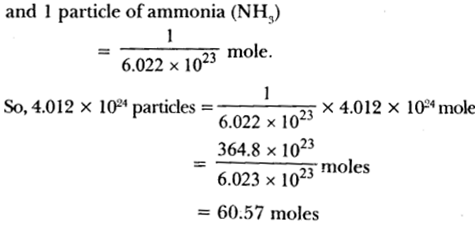Now, We also know that
1 mole NH3 contains = Molar mass of NH3
= (14 + 3 × 1)g = (14 + 3)g
= 17g
So, 6.66 moles of NH3 contains = (6.66 × 17)g = 113.25gQuestion 7.
(a) Define atomicity and poly atomic ions.
(b) Find out the atomicity of following:
CO2, NH3, S8, CaCO3, H2SO4, Ca(OH)2, K2SO4, Al2(SO4)3, NaCl.
(c) Write 2 divalent and 2 trivalent polyatomic ions.
(a) Atomicity: It is total number of atoms present in a molecule.
e,g.,

• Atomicity of H2 is 2.

Polyatomic ions: Ions which are formed from group of atoms are called polyatomic ions.
e,g.,

• Carbonate (CO32-), Sulphate (SO22-).

(b)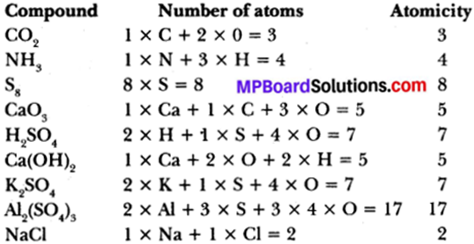(c) Divalent polyatomic ions:

• Carbonate ion (CO3s2-)
• Sulphate ion (SO42-).

Trivalent polyatomic ions:

• Phosphate ion (PO43-)
• Phosphite ion (PO33).

Atoms and Molecules Higher Order Thinking Skills (HOTS)

Question 1.
A thermos P contains 0.5 mole of oxygen gas. Another thermos Q contain 0.4 mole of ozone gas. Which of the two thermos contain greater number of oxygen atom?
1 molecule of oxygen (O2) = 2 atoms of oxygen
1 molecule of ozone (O3) = 3 atoms of oxygen
In thermos P:
1 mole of oxygen gas = 6.022 × 1023 molecules
0.5 mole of oxygen gas = 6.022 × 1023 × 0.5 molecules
= 6.022 × 1023 × 0.5 × 2 atoms
= 6.022 × 1023 atoms

In thermos Q:
1 mole of ozone gas = 6.022 × 1023 molecules
0.4 mole of oxygen gas = 6 .022 × 1023 × 0.4 molecules
= 6.022 × 1023 × 0.4 x 3 atoms
= 7.32 × 1023 atoms.

Question 2.
On analysing an impure sample of sodium chloride, the percentage of chlorine was found to be 45.5. What is the percentage of pure sodium chloride in the sample?
Molecular mass of pure NaCl
= Atomic mass of Na + Atomic mass of Cl
= 23 + 35.5 = 58.5u
Precentage of chlorine in pure NaCl
Now, if chlorine is 60.6 parts
NaCl =100 parts
If chlorine is 45.5 parts,
Thus, percentage of pure NaCl = 75%.

Question 3.
Write the chemical formulae of the following:

1. Ammonium phosphate
2. Iron sulphate
3. Calcium nitrate
4. Magnesium nitride
5. Ammonium sulphate
6. Aluminium chloride
7. Copper Nitrate
8. Aluminium sulphate
9. Sodium carbonate
10. Barium chloride
11. Calcium nitrate
12. Potassium chloride
13. Hydrogen sulphide
14. Magnesium hydroxide
15. Zinc sulphate.

1. Ammonium phosphate – (NH4)3PO4
2. Iron sulphate – -Fe2(SO4)3
3. Calcium nitrate – Ca(NO3)2
4. Magnesium nitrate – Mg(NO3)2
5. Ammonium sulphate – (NH4)2SO4
6. Aluminium chloride – AlCl3
7. Copper nitrate – CU(NO3)2
8. Aluminium sulphate – Al2(SO4)3
9. Sodium carbonate – Na2CO3
10. Barium chloride – BaCl2
11. Calcium nitrate – Ca(NO3)2
12. Potassium chloride – KCl
13. Hydrogen sulphide – H2S
14. Magnesium hydroxide – Mg(OH)2
15. Zinc sulphate – ZnSO4

Atoms and Molecules Value Based Question

Question 1.
Jolly buys gold ornaments and she is told that the ornaments has 90% gold and the rest is copper. She has been given a bill which amounts 100% charges of gold. Jolly refused to pay
the bill for 100% gold but settles the bill for 90% gold?
(a) How many atoms of gold are present in 1 gram of gold?
(b) Find out the ratio of gold and copper in the ornaments.
(c) What value of Jolly is seen in the above discussion?
(a) 1 gram of gold will contain $$\frac { 90 }{ 100 }$$ = 0.9 g of gold.